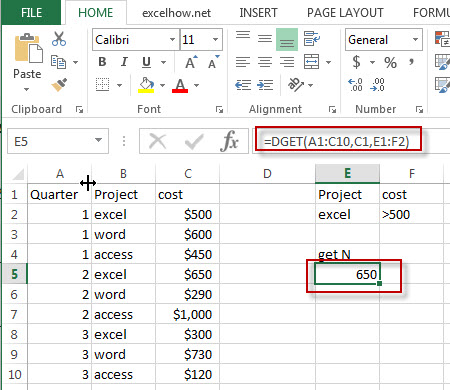# Excel Dget Function

This post will guide you how to use Excel DGET function with syntax and examples in Microsoft excel.

### Description

The Excel DGET returns a single value from a database that matches the specified conditions.

The DGET function is a build-in function in Microsoft Excel and it is categorized as a Database Function.

The DGET function is available in Excel 2016, Excel 2013, Excel 2010, Excel 2007, Excel 2003, Excel XP, Excel 2000, Excel 2011 for Mac.

### Syntax

The syntax of the DGET function is as below:

`= DGET(database, field, criteria)`

Where the DGET function arguments are:

• Database -This is a required argument.  The range of cells that containing the database.
• Field – This is a required argument.  The column to extract.
• Criteria – The range of cells that contains the conditions that you specify.

### Excel DGET Function Examples

The below examples will show you how to use Excel DGET Function to extract a single value from a column of a list or database that matches a given criteria.

#1 =DGET(A1:C10,C1,E1:F2)Note: The above excel formula will find excel project and  its cost is greater than 500, then return this cost value.